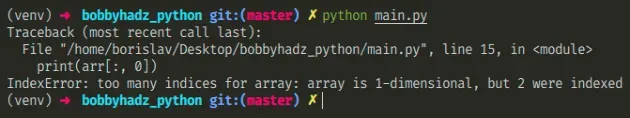# Solve - IndexError: too many indices for array in PythonFri Apr 22 20222 min read## Solve - IndexError: too many indices for array in Python#

The Python "IndexError: too many indices for array" occurs when we specify too many index values when accessing a one-dimensional numpy array. To solve the error, declare a two-dimensional array or correct the index accessor.Here is an example of how the error occurs.

main.py
```Copied!```import numpy as np

arr = np.array([1, 2, 3])

print(arr.shape) # 👉️ (3, )  👈️ this is one-dimensional array

# ⛔️ IndexError: too many indices for array: array is 1-dimensional, but 2 were indexed
print(arr[:, 0])
``````

We have a one-dimensional numpy array but specified 2 indexes which caused the error.

If you have a one-dimensional array, you can use a single index or a slice.

main.py
```Copied!```import numpy as np

arr = np.array([1, 2, 3])

print(arr)  # 👉️ 1
print(arr[0:2])  # 👉️ [1 2]
``````

You could declare a 2-dimensional numpy array instead.

main.py
```Copied!```import numpy as np

arr = np.array([[1, 2], [3, 4], [5, 6]])

print(arr.shape)  # 👉️ (3, 2) 👈️ this is two-dimensional array

print(arr[:, 0])  # 👉️ [1 3 5]
``````

The example above uses 2 indexes to get the first element of each nested array.

You can `print` the array you are trying to index to check whether you contains what you expect.

If you only have a one-dimensional array, use a single index when accessing it, e.g. `arr` or `arr[0:3]`.

Another common caused of the error is declaring a two-dimensional array where not all nested arrays have items of the same type and size.

main.py
```Copied!```import numpy as np

# 👇️ declared one-dimensional array (second nested list has only 1 item)
arr = np.array([[1, 2], , [5, 6]])

print(arr.shape)  # 👉️ (3,)

# ⛔️ IndexError: too many indices for array: array is 1-dimensional, but 2 were indexed
print(arr[:, 0])
``````

Notice that the second nested array only has 1 item, so we end up declaring a one-dimensional array.

A numpy array is an object that represents a multidimensional, homogenous array of fixed-size items.

If we add a second item to the second nested array, we would declare a two-dimensional array.

main.py
```Copied!```import numpy as np

arr = np.array([[1, 2], [3, 4], [5, 6]])

print(arr.shape)  # 👉️ (3, 2)

print(arr[:, 0])  # 👉️ [1 3 5]
``````

Notice that the shape of the array is `(3, 2)` as opposed to the array in the previous example which had a shape of `(3,)`.

Once you declare a two-dimensional array, you will be able to use two indices to access items in nested arrays.

main.py
```Copied!```import numpy as np

arr = np.array([[1, 2], [3, 4], [5, 6]])

# ✅ get the first item from the first two nested arrays
print(arr[0:2, 0])  # 👉️ [1 3]

# ✅ get the last item from the first two nested arrays
print(arr[0:2, -1])  # 👉️ [2 4]
``````
Use the search field on my Home Page to filter through my more than 1,000 articles.